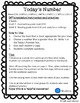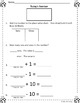# Number of the Day: Daily Routine for Mathematical Fluency in First GradeSubject
Resource Type
File Type

PDF

(2 MB|12 pages)
Standards
• Product Description
• StandardsNEW
This differentiated, open-ended, daily routine practices place value concepts (comparing numbers, tens & ones, ten more/less) & uses multiple representations of 2 digit numbers to build number sense.

Features:
•differentiated (2 levels)
•quick activity (10 minutes or less)
•comparing numbers
•place value (tens and ones)
•multiple representations of numbers (place value chart, base 10 blocks)
•ten more, ten less

Suggestions for Use:
Since it open-ended you can repeat it over and over with a new number each day. I often launch math time with this routine. You could use it for morning work or seatwork, leave it for substitutes, or send home for homework.

It provides a great spiral review for students to be practicing and using place value concepts all year.

For more engaging and differentiated activities, please check out:
•Place Value Find and Color No Prep Printables
Understand the meaning of the equal sign, and determine if equations involving addition and subtraction are true or false. For example, which of the following equations are true and which are false? 6 = 6, 7 = 8 - 1, 5 + 2 = 2 + 5, 4 + 1 = 5 + 2.
Subtract multiples of 10 in the range 10-90 from multiples of 10 in the range 10-90 (positive or zero differences), using concrete models or drawings and strategies based on place value, properties of operations, and/or the relationship between addition and subtraction; relate the strategy to a written method and explain the reasoning used.
Given a two-digit number, mentally find 10 more or 10 less than the number, without having to count; explain the reasoning used.
Compare two two-digit numbers based on meanings of the tens and ones digits, recording the results of comparisons with the symbols >, =, and <.
Understand that the two digits of a two-digit number represent amounts of tens and ones. Understand the following as special cases:
Total Pages
12 pages
Included
Teaching Duration
N/A
Report this Resource to TpT
Reported resources will be reviewed by our team. Report this resource to let us know if this resource violates TpT’s content guidelines.